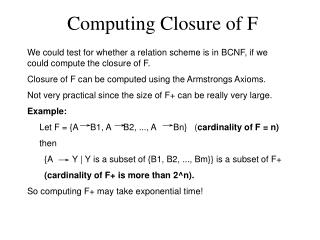# Computing Closure of F - PowerPoint PPT PresentationDownload PresentationComputing Closure of F

Computing Closure of F
Download Presentation## Computing Closure of F

- - - - - - - - - - - - - - - - - - - - - - - - - - - E N D - - - - - - - - - - - - - - - - - - - - - - - - - - -
##### Presentation Transcript

1. Computing Closure of F We could test for whether a relation scheme is in BCNF, if we could compute the closure of F. Closure of F can be computed using the Armstrongs Axioms. Not very practical since the size of F+ can be really very large. Example: Let F = {A B1, A B2, ..., A Bn} (cardinality of F = n) then {A Y | Y is a subset of {B1, B2, ..., Bm}} is a subset of F+ (cardinality of F+ is more than 2^n). So computing F+ may take exponential time!

2. Membership of F+ Fortunately, to test for BCNF we do not need to compute closure of F. Instead we only need to test if a dependency X Y is in F+ Testing for membership in F+ can be done efficiently. To develop an algorithm for testing membership in F+, we need to define the notion of a closure of a set of attributes Closure of attribute set: Let R be a relation scheme and F be the functional dependency set. Closure of a set of attributes X with respect to F denoted by X+ is the set of attributes Ai of R such that X Ai can be derived using Armstrong Axioms. Note: X Y holds over R if and only if Y is a subset of X+.

3. Membership of F+ Since X Y holds over R if and only if Y is a subset of X+, we can check if X Y holds by computing X+ and testing if Y is a subset of X+. Hence X Y is a element of F+ if and only if Y is a subset of X+ Computing X+ X+ = X repeat oldX+ = X+ for each fd Y Z in F do if (Y is a subset of oldX+) then X+ = X+ union Z endif endfor until (oldX+ == X+) maximum number of iterations = cardinality of F times the number of attributes in R! (polytime)

4. Example • Let the set F contain the following fds: • AB C, D EG, C A, BE C, BC D • CG BD, ACD B, CE AG Let X = BD. Compute X+. iteration 1: X+ = {BD} iteration 2: X+ = {BDEG} (due to dependency 2) iteration 3: X+ = {BDCEG} (due to dependency 3) iteration 4: X+ = {BCDEGA} (due to dependency 8) iteration 5: X+ = {BCDEGA} Algorithm exits the loop since no new attribute added in last iteration and (BD)+ = {ABCDEG}